### Before you start

This post is about how to create your themes and palettes in Grammar of Graphics 2 (ggplot2). I assume you know what ggplot2 is and have a basic understanding of its functionality. I also assume you’ve used ggplot2 before but if you did not, you can watch the fantastic two parts webinar by Thomas Lin Pedersen. The first part is here and the second is here. I honestly think that it provides a very good overview of what ggplot2 is all about. To learn more about themes, please visit the ggplot2 help page, here. Finally, I assume you know how to create and use functions in R.

Firstly, I’ll need to load the necessary packages. I will be using `ggplot2`, `colorspace`, `viridis`, `ggcorrplot`, `magrittr`, and (at the time of writing this post) new package called `ggpattern` that I’ve been looking forward to.

You can see the required packages in the snippet below. Except for `ggpattern`, all can be downloaded from CRAN. You’ll need to install the `ggpattern` using `remotes::install_github("coolbutuseless/ggpattern")`.

``````library(ggplot2)
library(colorspace)
library(viridis)
library(ggpattern)
library(ggcorrplot)
library(magrittr)``````
``##  '3.3.0'``
``##  '1.4.1'``
``##  '0.5.1'``
``##  '0.1.0'``
``##  '0.1.3'``
``##  '1.5'``

The theme I will be developing here is aiming for simplicity but this doesn’t mean yours can’t be a fancy one. Whichever style you are going for, I suggest you start building the theme from one of the default ggplot2 themes. The theme I am doing is build on `theme_minimal()` - a minimalistic theme that relies a lot on `element_blank()` function (which hides a given element in theme).

Let’s look at the theme_minimal() below:

``theme_minimal # Print the theme in console without ().``
``````## function (base_size = 11, base_family = "", base_line_size = base_size/22,
##     base_rect_size = base_size/22)
## {
##     theme_bw(base_size = base_size, base_family = base_family,
##         base_line_size = base_line_size, base_rect_size = base_rect_size) %+replace%
##         theme(axis.ticks = element_blank(), legend.background = element_blank(),
##             legend.key = element_blank(), panel.background = element_blank(),
##             panel.border = element_blank(), strip.background = element_blank(),
##             plot.background = element_blank(), complete = TRUE)
## }
## <bytecode: 0x0000000017e13300>
## <environment: namespace:ggplot2>``````

First, you’ll see that the theme is using an additional theme inside of its function. Specifically, `theme_bw()` which in turn uses `theme_gray()` which when you call prints about 60 rows of code build inside the `theme()`. That is the function you will use to develop the theme.

To develop and customise the theme, you need to define a new function and copy the arguments inside theme_minimal(). The code below shows the final theme and how I’ve customised it:

``````project_theme <- function( # Define function and its arguments
base_text_size = 15, # Set overall size of text elements
base_text_axis_x_size = 12, # Set size of text on x-axis
base_text_axis_x_angle = 90, # Set angle of x-axis text
base_text_axis_x_hjust = 1, # Make sure to keep the text in center after adjusting angle
base_text_axis_x_vjust = 0.5 # vjust stands for vertical adjustment, hjust for horizontal
) {

# Define the theme.
# In my case, I am  hiding lots of the elements in order to create a simple looking plot.
# The elements I do need to control are defined with arguments, which will need to be set inside the function.
# I also don't want to set the arguments every time I call them, so I've created default values.

theme(axis.ticks = element_blank(),
# The part before "=" determines what element of plot will be modified.
# The part after "=" determines what to do with the element. In this case, I'll hide it.
legend.background = element_blank(),
legend.key = element_blank(),
panel.background = element_blank(),
panel.border = element_blank(),
strip.background = element_blank(),
plot.background = element_blank(),
text = element_text(size = base_text_size), # Arguments for function:
axis.text.x = element_text(size = base_text_axis_x_size,
angle = base_text_axis_x_angle,
hjust = base_text_axis_x_hjust,
vjust = base_text_axis_x_vjust))
}``````

Now the theme is going to behave as any other function and you can reuse it for all your plots.

Option 1 is to use your theme every time you create a plot, like this:

``````ggplot(data = cars, aes(x = dist, y = speed)) +
geom_point() +
project_theme()``````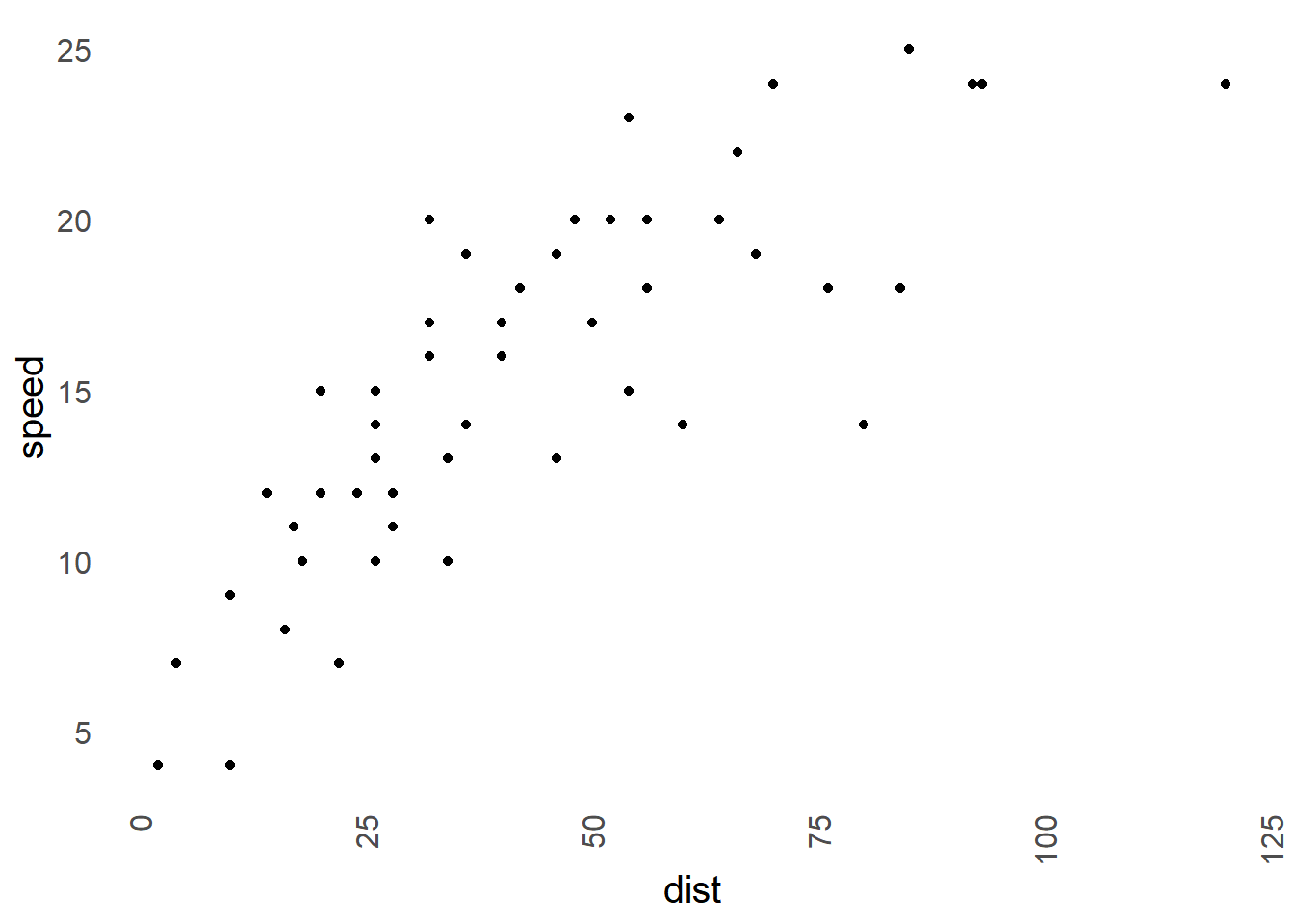Option 2 is to set the theme using ggplot function `theme_set()`. Then any plot you create will be using the set theme. This is preferred when you need to do a lot of visualisations as typing `theme_set()` every time is somewhat tiring.

``````theme_set(project_theme()) # Set the theme.

ggplot(data = cars, aes(x = dist, y = speed)) +
geom_point()``````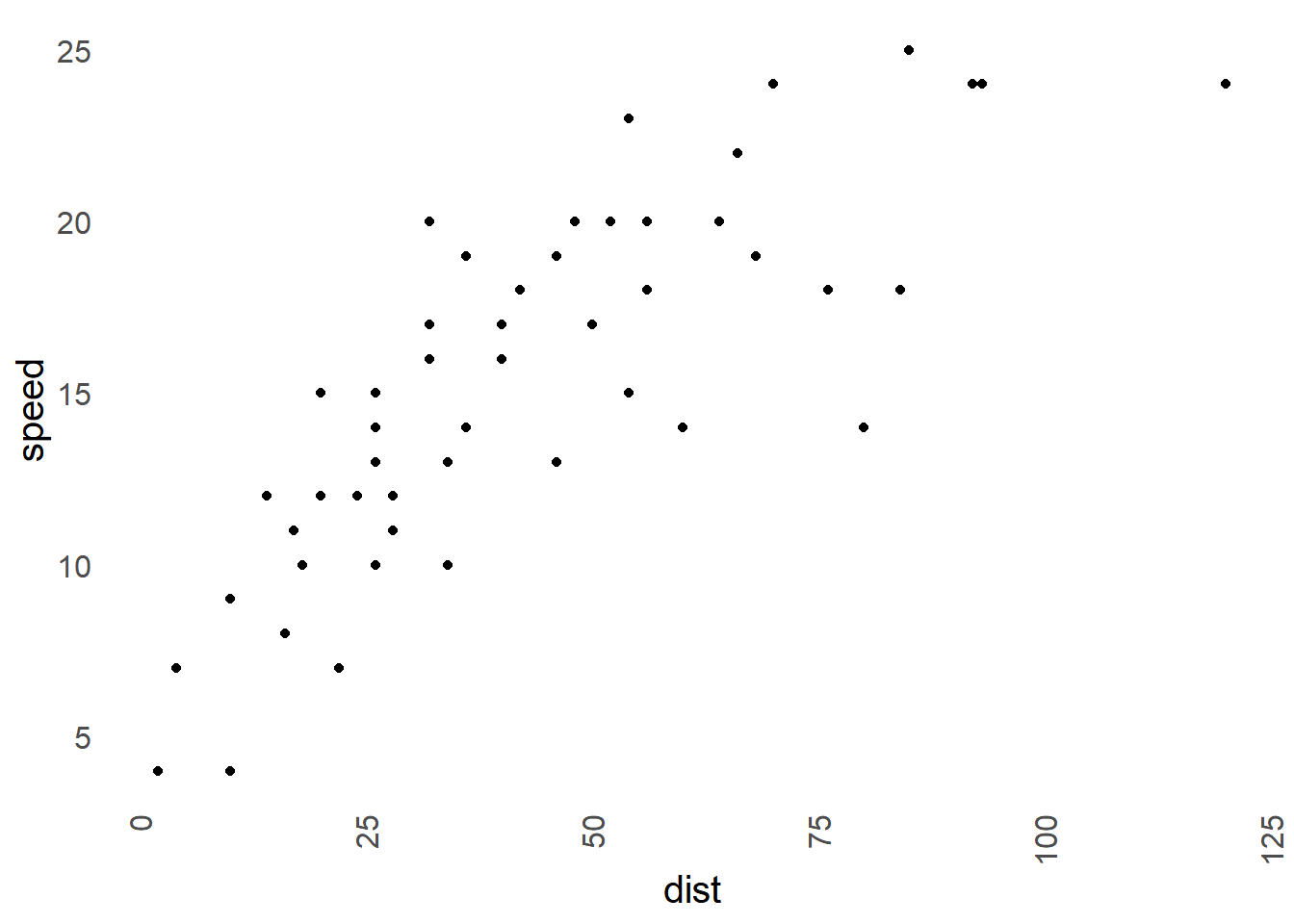``theme_set(theme_gray()) # Set the theme back to default ggplot2 theme. ``

Besides `theme_set()`, you can also use other functions (click here) that ease the application of theme. Specifically, `theme_get()`, `theme_update(...)`, `theme_replace(...)`. I invite you to explore the help page yourself.

Unfortunately, the `theme_set()` does not seem to affect plots created by packages relying on ggplot2; however, in most cases, you can still use `+ theme()` and modify these plots:

``````# The default output from ggcorrplot()
ggpre <- cars %>%
cor() %>%
ggcorrplot() +
ggtitle("Pre")

# Using theme_update
ggpost <- cars %>%
cor() %>%
ggcorrplot() +
theme(
axis.text.x = element_text(angle = 90, vjust = 0.5, size = 15, hjust = 0.5, colour = "blue"),
axis.text.y = element_text(angle = 90, vjust = 0.5, size = 15, hjust = 0.5, colour = "blue")
) +
ggtitle("Post")

gridExtra::grid.arrange(ggpre, ggpost, ncol = 2)``````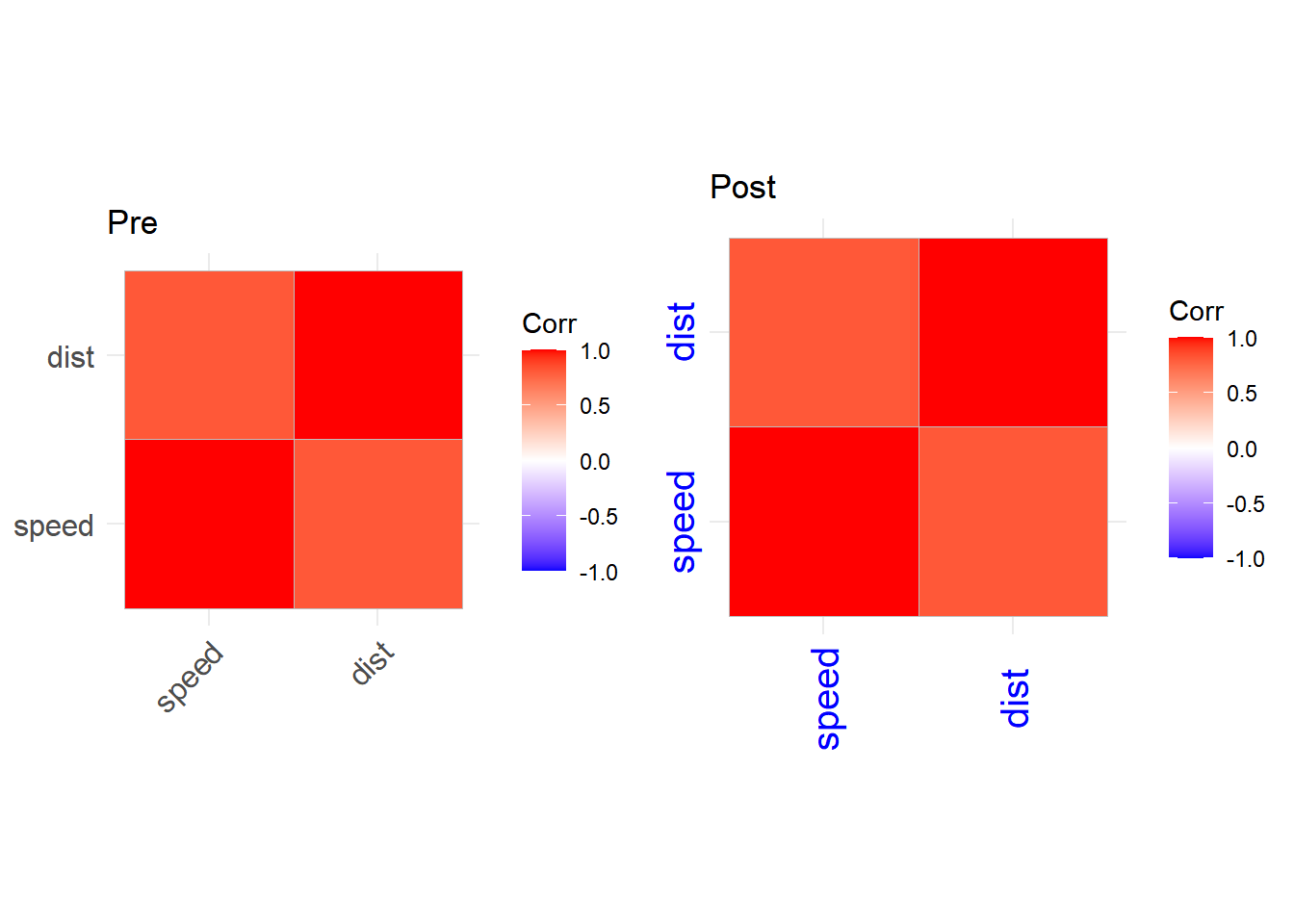In every project, it’s good to use specific palettes to ensure colours are being used consistently across all your plots. I’ll assume that you know about how ggplot2 understands the colours or you can read on that here if you want.

Colours clearly make much more interesting plots and ease the interpretation. However, they should be used with caution as they can also mislead the interpretation if they are used incorrectly. Therefore, the best place to start using them is to use one of the pre-defined palettes. Luckily, ggplot2 has plenty of such palettes and they are good if you don’t even want to install an additional package. I think you can’t go wrong with either viridis or color brewer 2.

Viridis is used for continuous scales and you can use viridis like this in ggplot2:

``````ggplot(data = cars, aes(x = dist, y = speed)) +
geom_point(aes(size = speed, colour = speed)) +
scale_colour_viridis_c(option = "D") + # _c is for continuous; "D" is for palette option
project_theme() + # I use the project theme I've defined above
guides(colour = "legend")``````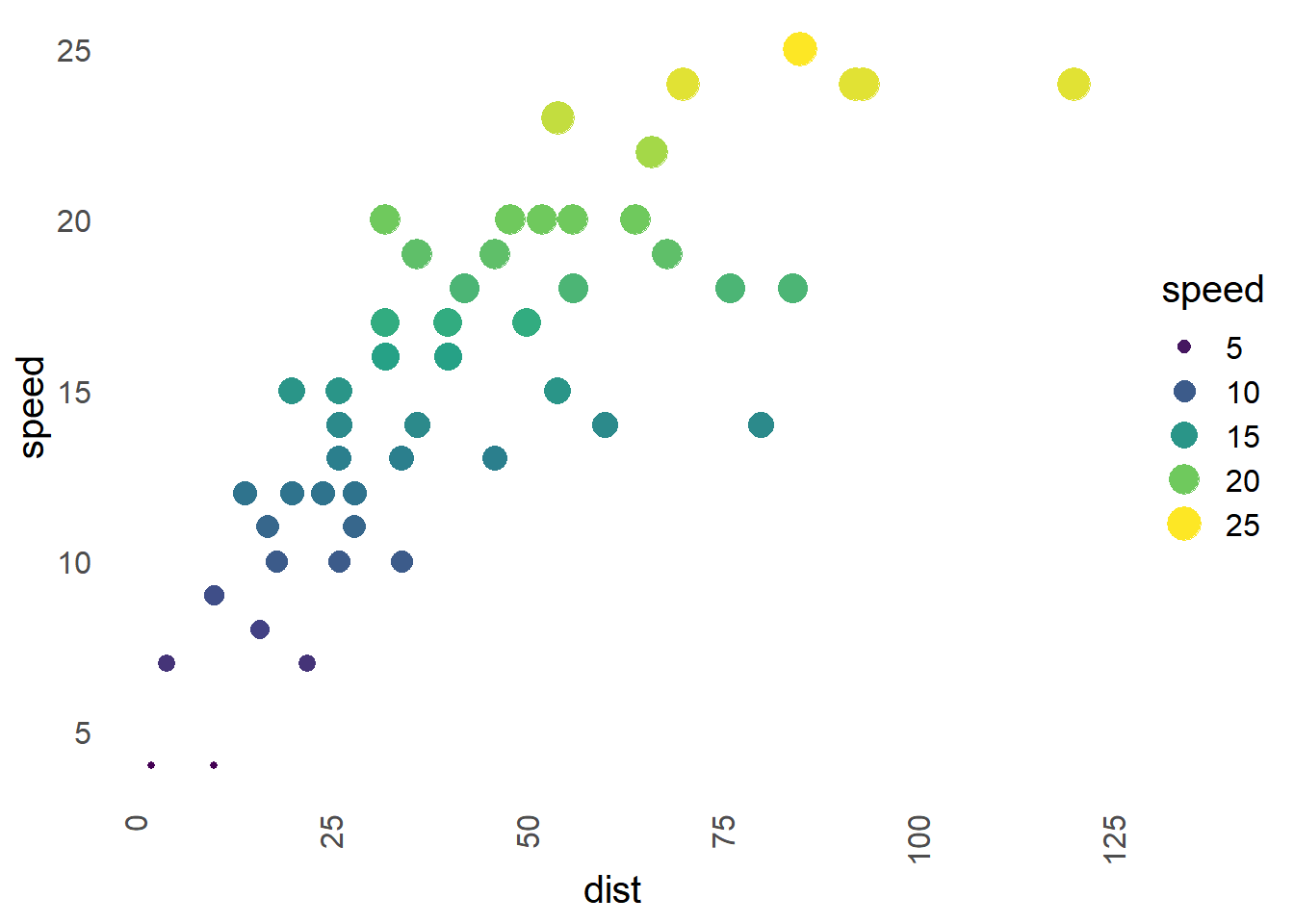Brewer is used for discrete scales and to use it color brewer, do this:

``````cars\$speed2 <- factor(cut(cars[, "speed"], 3)) # Since brewer doesn't handle discrete scales, you need to convert any continuous variables to factor!

ggplot(data = cars, aes(x = dist, y = speed)) +
geom_point(aes(size = speed2, colour = speed2)) +
scale_color_brewer(type = "seq", palette  = "RdPu") + # Seq is for sequential; "RdPu" is a palette type
project_theme() + # I use the project theme I've defined above
guides(colour = "legend") # I used both size and colour, this merges the legend.``````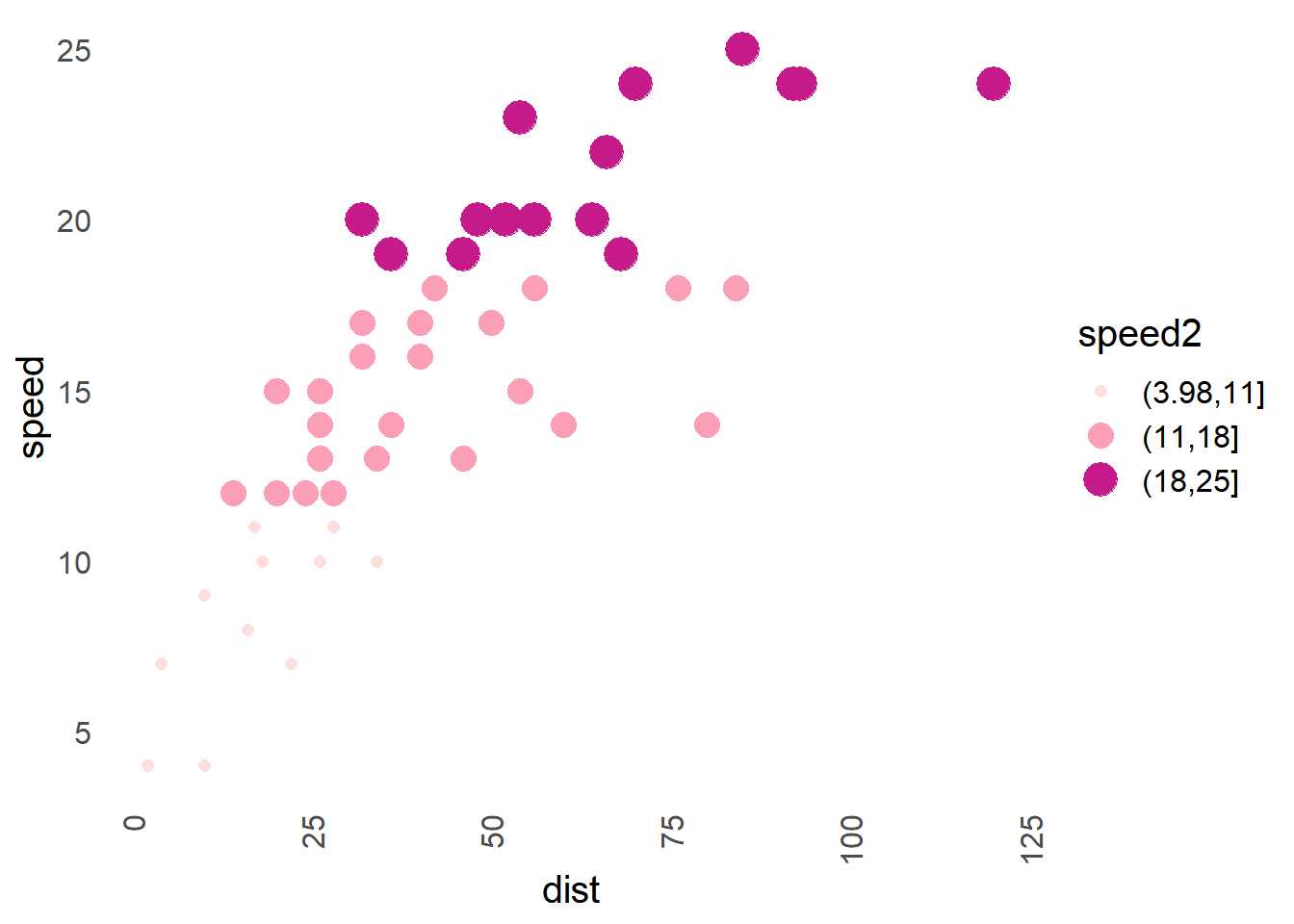Let’s create a custom palette. I’ll be using `colorspace` package for this and my aim here will be to create a grey palette that can be used for your boring academic journals as most of them do hate colours. Before I’ll illustrate how I create my palette, I’d suggest exploring pre-defined palettes as they might be what you are looking for.

Brewer offers one such palette, namely “Greys”. You can use it like this:

``````cars\$speed2 <- factor(cut(cars[, "speed"], 3))

ggplot(data = cars, aes(x = dist, y = speed)) +
geom_point(aes(size = speed2, colour = speed2)) +
scale_color_brewer(palette = "Greys") + #"Greys" is for a palette type
project_theme() +
guides(colour = "legend")``````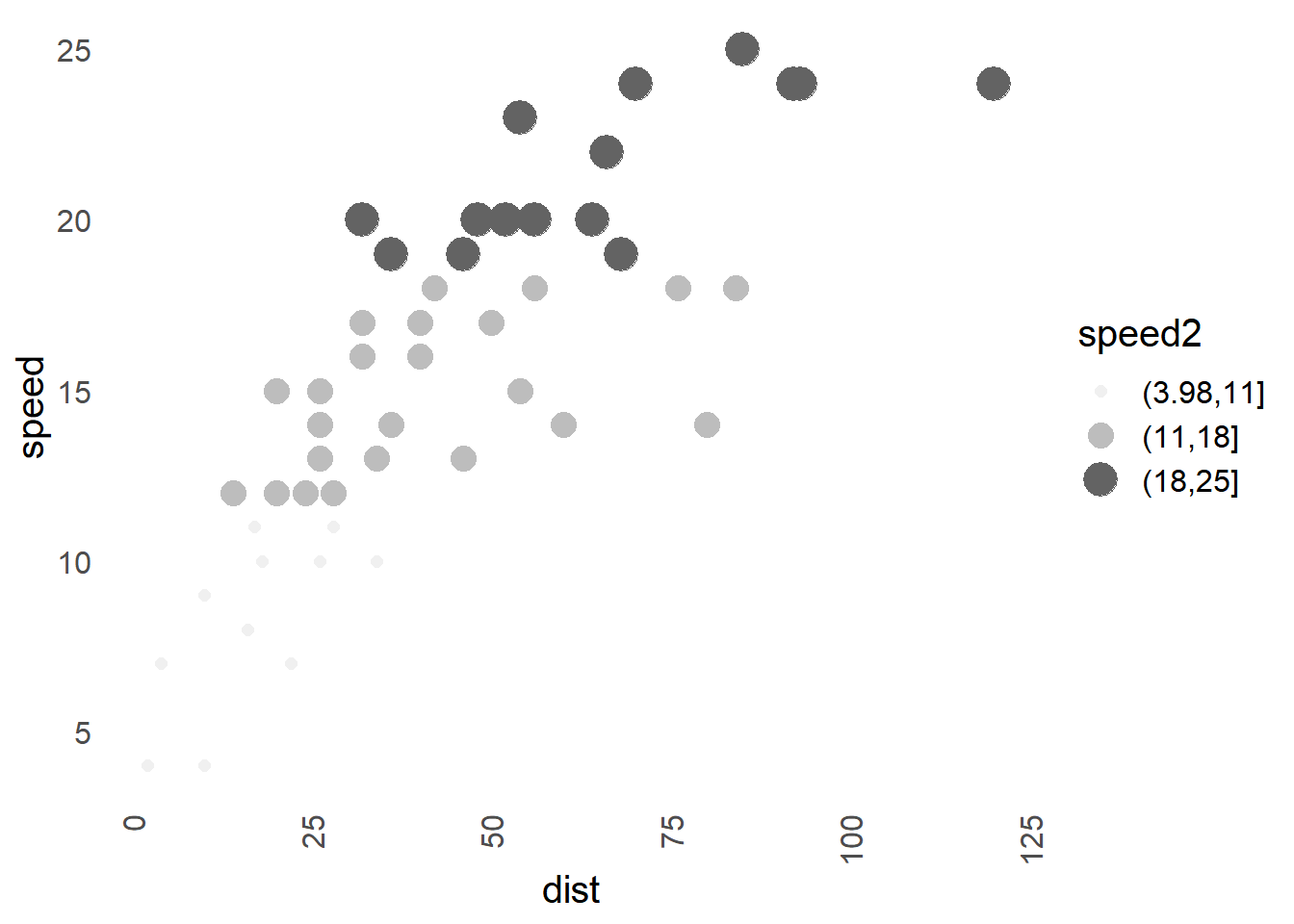There are a few problems here. Firstly, I use a white background and you can’t see the light grey very well. Second, the brewer is meant to be used for discrete scale and this makes it a bit annoying to use if you work with continuous scale. Now enter the `colorspace` to save the day!

Let’s first talk about “Why?” `colorspace` package. There are several reasons: 1. It is versatile and can be used for both discrete and continuous scales. 2. It provides functions that allow you to experiment and test the palettes before you apply them. 3. It can do all that brewer does and more. 4. The package is very well documented (vignettes, tutorials, shiny apps). 5. Not limited to R only.

Firstly, there are two palettes in `colorspace`, specifically, `hcl_palettes(n = 7, palette = "Light Grays", plot = TRUE)` and `hcl_palettes(n = 7, palette = "Grays", plot = TRUE)`. The chances are that these are what you are looking for, they look like this in plots:

``````cars\$speed2 <- factor(cut(cars[, "speed"], 3))

gg1 <- ggplot(data = cars, aes(x = dist, y = speed)) +
geom_point(aes(size = speed2, colour = speed2)) +
scale_color_discrete_sequential(palette = "Light Grays") + # Similar to color brewer
project_theme() +
guides(colour = "legend") +
ggtitle("Light Grays - discrete")

gg2 <- ggplot(data = cars, aes(x = dist, y = speed)) +
geom_point(aes(size = speed2, colour = speed2)) +
scale_color_discrete_sequential(palette = "Grays") + # Similar to color brewer
project_theme() +
guides(colour = "legend") +
ggtitle("Grays - discrete")

gg3 <- ggplot(data = cars, aes(x = dist, y = speed)) +
geom_point(aes(size = speed, colour = speed)) +
scale_color_continuous_sequential(palette = "Light Grays") + # Similar to color brewer
project_theme() +
guides(colour = "legend") +
ggtitle("Light Grays - continuous")

gg4 <- ggplot(data = cars, aes(x = dist, y = speed)) +
geom_point(aes(size = speed, colour = speed)) +
scale_color_continuous_sequential(palette = "Grays") + # Similar to color brewer
project_theme() +
guides(colour = "legend") +
ggtitle("Grays - continuous")

gridExtra::grid.arrange(gg1, gg2, gg3, gg4, nrow = 2, ncol = 2)``````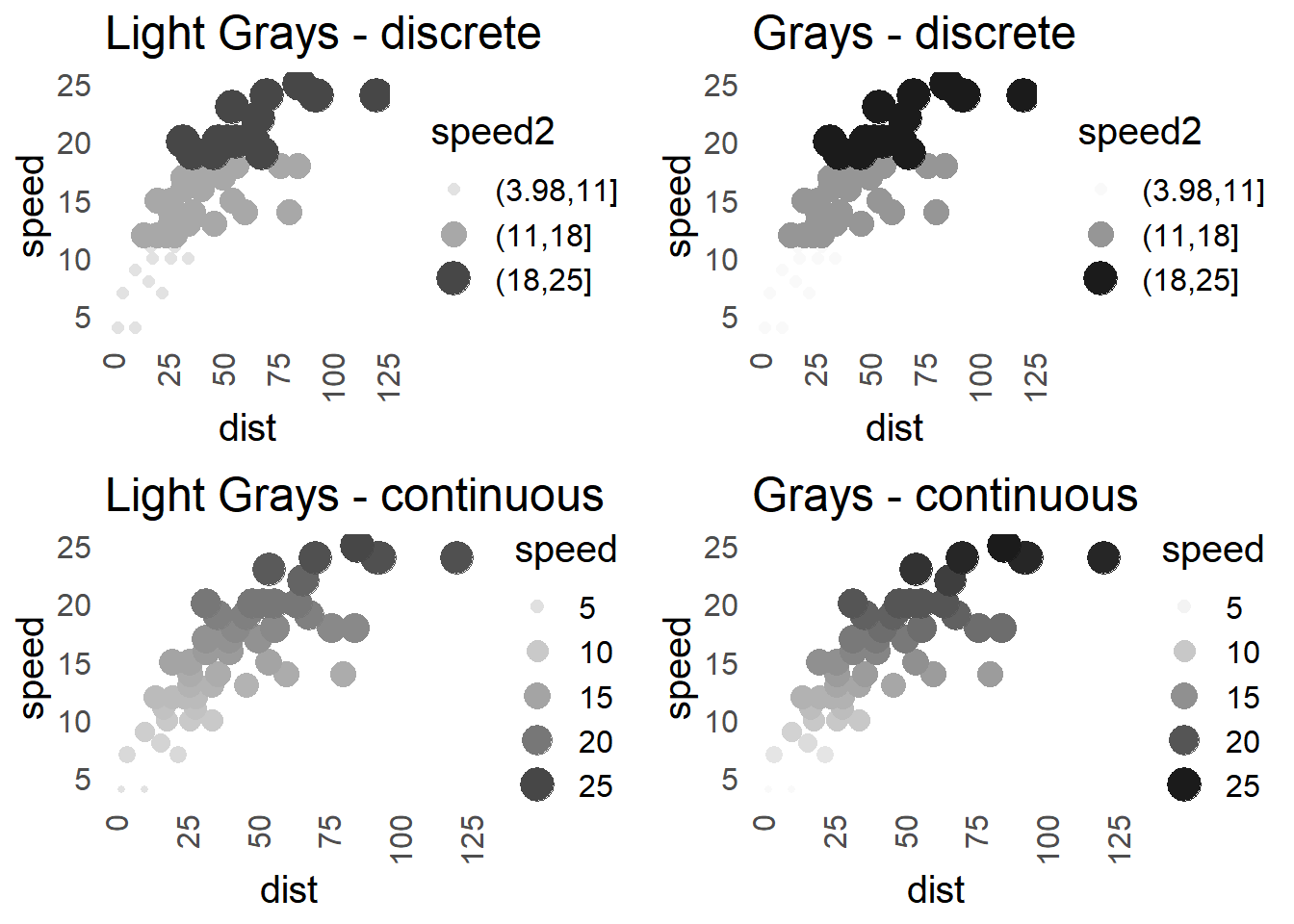Chances are you are happy with these; however if you still want to create your own - read further.

To start creating a palette, I’d urge you to either call `hcl_wizard()` in console or visit the corresponding page where you can use the “Palette Creator”. You then can explore available palettes and customise them. There is so much documentation available that I won’t cover the process, it is also quite an intuitive app to use. In short, the `hcl_wizard` takes you to an app with various sliders which you can adjust to create the palette. Once you are done, you’ll go to “Export” page and select “R” tab. You’ll see (depending on what you’ve created) something like this:

`qualitative_hcl(n = 7, h = c(30, -330), c = 50, l = 70, register = )`

In my case, I’ve been trying to create grey palette (you can see such palettes under "Basic: Sequential (single-hue)) that doesn’t rely on white at the end of one coulor spectrum because I use a white background in my theme I also wanted to avoid light grey and pick fewer (n = 5) but distinguishable shades of colour. I’ve proceed to create it in `hcl_wizard()` and tried it on couple of my plots, this is the result:

``````palette_gray_mc <- colorspace::sequential_hcl(n = 5, h = c(-360, -360),
c = c(0, 0, 0), l = c(20, 85),
power = c(0, 1), fixup = FALSE,
register = "palette_gray_mc")``````

You’ll also see that I’ve used the “register” option of my palette, but also saved it in vector. The reason for saving it in the vector is that I often need to use only one of the colours. So, I save the individual colours like this:

``````# Color from palette above pal_gray_mc.
color_e <- "#D4D4D4"
color_d <- "#A8A8A8"
color_c <- "#7D7D7D"
color_b <- "#555555"
color_a <- "#303030"``````

Finally, to use the palette, I can either pick a colour and use it outside aes() of ggplot, e.g.: `color = color_a`, but since I’ve registered the palette, the proper way is to do this:

``````ggplot(data = cars, aes(x = dist, y = speed)) +
geom_point(aes(size = speed, colour = speed)) +
scale_color_continuous_sequential(palette = "palette_gray_mc") + # Use it like this!
project_theme() +
guides(colour = "legend") +
labs(title = "Martin's awesome grey palette!",
caption = "Okay, maybe.")``````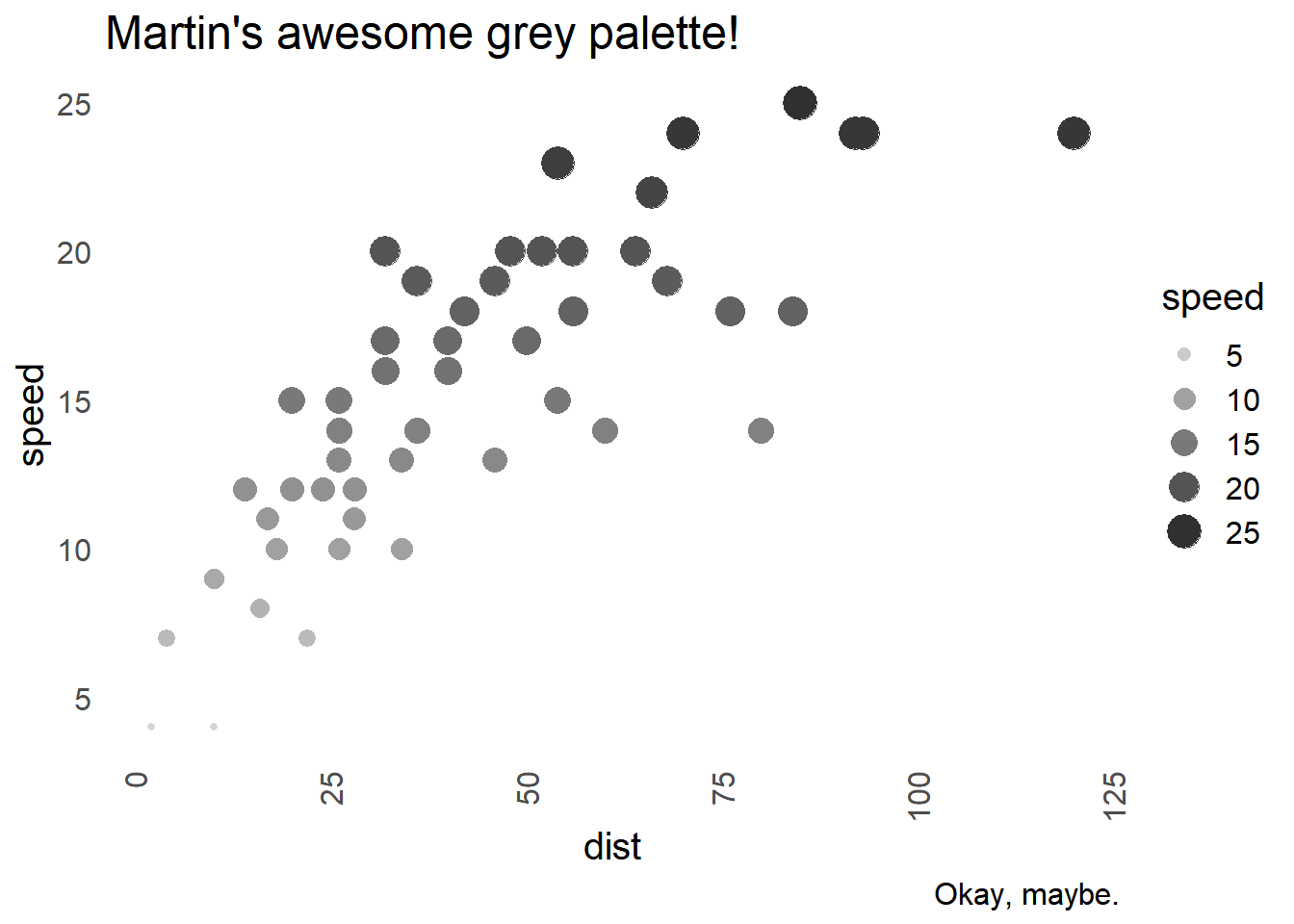### When even shades of grey fail you

Sometimes I feel that no matter what shade of grey I use, it’s no good. However, that’s where ggpattern comes to play. I won’t talk about the package much as it’s still an early release and may change a lot in the future, but I think a lot of people may benefit from using it.

Using the cars dataset, thanks to the ggpattern, I can plot the speed in a bar chart like this:

``````cars\$speed2 <- factor(cut(cars[, "speed"], 3))  # Let's use our modified cars dataset

ggplot(data = cars) +
geom_bar_pattern(
aes(speed2, pattern = speed2, pattern_angle = speed2),
pattern = "stripe",
fill    = "white",
colour  = "black") +
project_theme() +
theme(legend.position = 'none') +
labs(x = "Speed (factor)", y = "Frequency")``````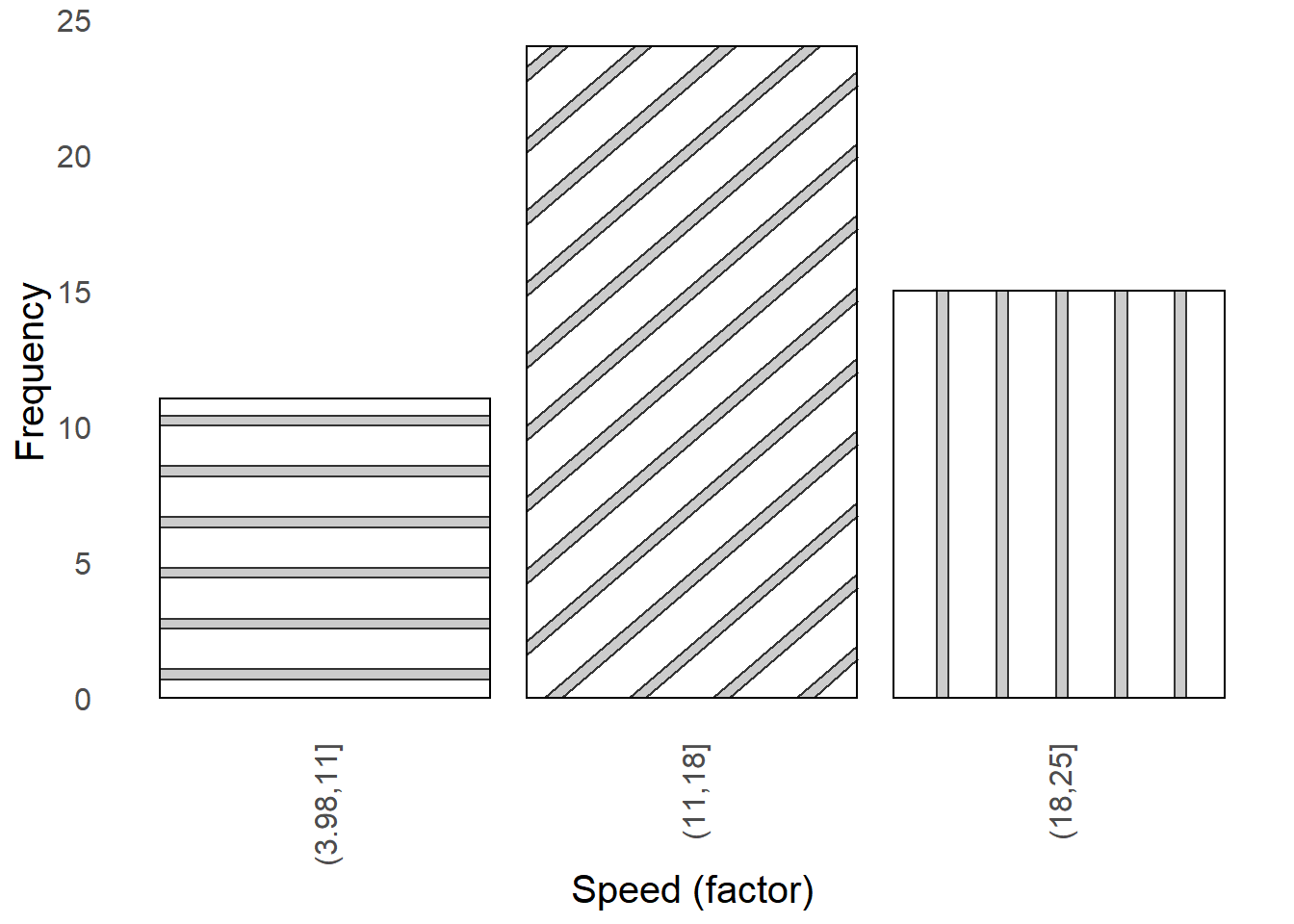This is useful as I don’t have to scratch my head around which shade of grey is different from other shade of grey (trust me, it’s sometimes impossible to tell).

#### Thank you

Thank you very much for reading the post and I hope you’ve found it useful. I have not been posting at all but I want to start again. Since it takes some time to create a post like this, any feedback or interest will help me to continue doing this (especially if you liked it and found it useful).